### Rankine plasticity model with isotropic damage and its nonlocal formulations - RankMat, RankMatNl, RankMatGrad

This model has a very similar structure to the model described in Section 1.4.3, but is based on the Rankine yield condition. It is available in the small-strain version only, and so far exclusively for plane stress analysis. The basic equations (38)-(39) and (41)-(45) remain valid, and the yield function (40) is redefined as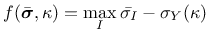(53)

where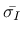are the principal values of the effective stress tensor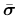. The hardening law can have either the linear form (42), or the exponential form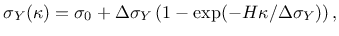(54)

where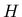is now the initial plastic modulus and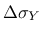is the value of yield stress increment asymptotically approached as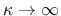. In damage law (45), parameter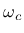is always set to 1. If the plastic hardening is linear, the user can specify either the exponent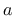from (45), or the dissipation per unit volume,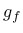, which represents the area under the stress-strain diagram (and parameteris then determined automatically such that the area under the diagram has the prescribed value). For exponential plastic hardening, the evaluation offromis not properly implemented and it is better to specifydirectly.

The model description and parameters are summarized in Tabs. 13-15. Note that the default value of parameter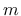is equal to 1 for the integral model but for the gradient model it is equal to 2. Also note that the internal length parameter r has the meaning of the radius of interactionfor the integral version (and thus has the dimension of length) but for the gradient version it has the meaning of the coefficient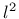multiplying the Laplacean in (51), and thus has the dimension of length squared.

For the gradient model it is possible to specify parameter negligible_damage, which sets the minimum value of damage that is considered as nonzero. The approximate solution of Helmholtz equation (51) can lead to very small but nonzero nonlocal kappa at some points that are actually elastic. If such small values are positive, they lead to a very small but nonzero damage. If this is interpreted as "loading", the tangent terms are activated, but damage will not actually grow at such points and the convergence rate is slowed down. It is better to consider such points as elastic. By default, negligible_damage is set to 0, but it is recommended to set it e.g. to 1.e-6.

Table 13: Rankine plasticity - summary.
 Description Rankine plasticity with isotropic hardening and damage Record Format RankMat (in) # d(rn) # E(rn) # n(rn) # plasthardtype(in) # sig0(rn) # H(rn) # delSigY(rn) # yieldtol(rn) # (gf(rn) #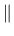a(rn) #) Parameters - material number - d material density - E Young's modulus - n Poisson's ratio - plasthardtype type of plastic hardening (0=linear=default, 1=exponential) - sig0 initial yield stress in uniaxial tension (compression) - H initial hardening modulus (default value 0.) - delSigY final increment of yield stress (default value 0., needed only if plasthardtype=1) - yieldtol relative tolerance in the yield condition - gf dissipation per unit volume - a exponent in damage law (45) Supported modes PlaneStress

Table 14: Nonlocal integral Rankine plasticity - summary.
Description Nonlocal Rankine plasticity with isotropic hardening and damage
Record Format RankMatNl (in) # d(rn) # E(rn) # n(rn) # plasthardtype(in) # sig0(rn) # H(rn) # delSigY(rn) # yieldtol(rn) # (gf(rn) #a(rn) #)r(rn) #m(rn) #[wft(in) #][scalingType(in) #]
Parameters - material number
- d material density
- E Young's modulus
- n Poisson's ratio
- plasthardtype type of plastic hardening (0=linear=default, 1=exponential)
- sig0 initial yield stress in uniaxial tension (compression)
- H initial hardening modulus (default value 0.)
- delSigY final increment of yield stress (default value 0.)
- yieldtol relative tolerance in the yield condition
- gf dissipation per unit volume
- a exponent in damage law (45)
- r internal length scale parameterfrom eq. (51)
- m over-nonlocal parameter (default value 1.)
- wft selects the type of nonlocal weight function (see Section 1.5.7):
 1 - default, quartic spline (bell-shaped function with bounded support) 2 - Gaussian function 3 - exponential function (Green function in 1D) 4 - uniform averaging up to distance5 - uniform averaging over one finite element 6 - special function obtained by reducing the 2D exponential function to 1D (by numerical integration)
- scalingType selects the type of scaling of the weight function (e.g. near a boundary; see Section 1.5.7):
 1 - default, standard scaling with integral of weight function in the denominator 2 - no scaling (the weight function normalized in an infinite body is used even near a boundary) 3 - Borino scaling (local complement)
Supported modes PlaneStress

Table 15: Gradient-enhanced Rankine plasticity - summary.
 Description Gradient-enhanced Rankine plasticity with isotropic hardening and damage Record Format RankMatGrad (in) # d(rn) # E(rn) # n(rn) # plasthardtype(in) # sig0(rn) # H(rn) # delSigY(rn) # yieldtol(rn) # (gf(rn) #a(rn) #)r(rn) #m(rn) #negligible_damage(rn) # Parameters - material number - d material density - E Young's modulus - n Poisson's ratio - plasthardtype type of plastic hardening (0=linear=default, 1=exponential) - sig0 initial yield stress in uniaxial tension (compression) - H hardening modulus (default value 0.) - delSigY final increment of yield stress (default value 0.) - yieldtol relative tolerance in the yield condition - gf dissipation per unit volume - a exponent in damage law (45) - r internal length scale parameter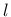from eq. (51) - m over-nonlocal parameter (default value 2.) - negligible_damage optional parameter (default value 0.) Supported modes PlaneStress

Borek Patzak
2019-03-19